# Array Abstract Data Type

## Array as ADT (Abstract Data Type) using C and C++ Language:

In this article, I am going to discuss Array as ADT (Abstract Data Type) using C and C++ Language with Examples. What do we mean by ADT here? ADT means –

1. Representation of Data.
2. Set of Operations on the Data.

C/C++ provides Array as a basic data structure. Representation of data is defined by the language compiler itself. But the operations on the data are not defined by the compiler. We have to implement or provide the operations on Array data structure. So, data structure array and the set of operations together we can call it as Abstract Data Type (ADT).

##### Operations on Array Data Structure:

Below is the list of some of the operations that we can perform on an array-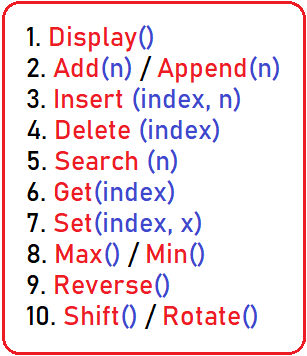We can perform more operations on the array data structure but the above are some basic operations. Let us give some information about the above functions-

1. Display () – To Display the entire array on the screen.
2. Add(n) / Append(n) – To add a particular element on the end of the array.
3. Insert (index, n) – To add an element to a particular index.
4. Delete (index) – To delete an element with the help of an index in the given array.
5. Search (n) – To check whether the given element is present or not in an array.
6. Get (index) – It will return the element which presents on the given index.
7. Set (index, x) – It will change the element with the new element at a particular index.
8. Max () / Min () – These will return the max and min element in the given array.
9. Reverse () – It will reverse the order of elements in the given array.
10. Shift () – It will shift the whole elements either on the left or right side by the given number.
##### Representation of Data on Array:

Now let’s look at the representation of the data on an array.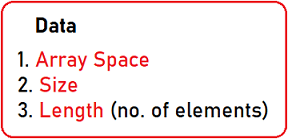The first thing is we need an array space of some size. Here we initialize an array of size = 10 and length = 0 because there are no elements in the array.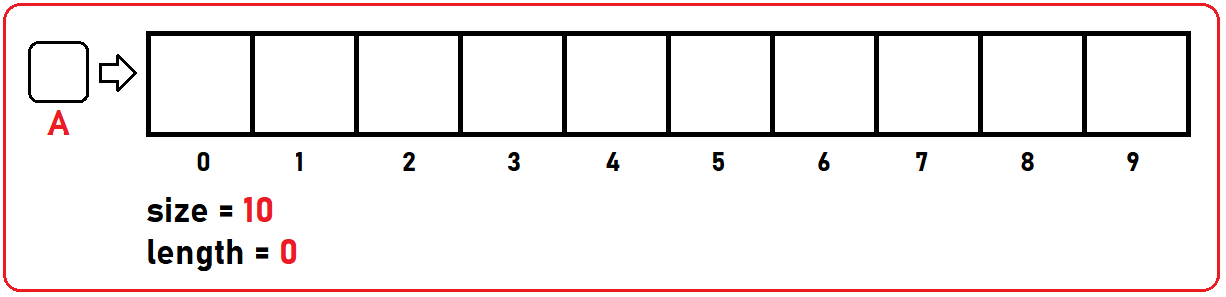Here we have two methods to initialize our Array:

1. Inside Stack Memory: As shown in the below image, A  will create a contiguous block of memory index from 0 to 9 inside Stack.
2. Inside Heap Memory: We can also create our array dynamically with the new keyword, The new operator denotes a request for memory allocation, as shown in the below image, we created a pointer of int* type and in the next step we point it to a contiguous block of memory inside Heap. We have created our array inside heap memory by using a pointer.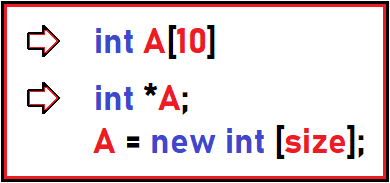##### The Complete C Code:
```#include <stdio.h>
#include <stdlib.h>

struct Array {
int* A;
int size;
int length;
};

int main() {
struct Array arr;

printf("Enter Size of an Array: ");
scanf("%d", &arr.size);

arr.A = (int*)malloc(sizeof(int) * arr.size);
arr.length = 0;

printf("Enter Number of Elements: ");
scanf("%d", &arr.length);

printf("Enter All Elements: \n");
for (int i = 0; i < arr.length; i++) {
scanf("%d", &arr.A[i]);
}

getchar();
}
```
###### Output: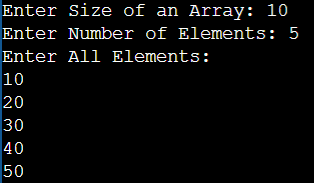##### The Complete C++ Code:
```#include <iostream>
using namespace std;

struct Array {
int* A;
int size;
int length;
};

int main() {
struct Array arr;

cout << "Enter Size of an Array: " << endl;
cin >> arr.size;

arr.A = new int[arr.size];
arr.length = 0;

cout << "Enter Number of Elements: " << endl;
cin >> arr.length;

cout << "Enter All Elements: " << endl;
for (int i = 0; i < arr.length; i++) {
cin >> arr.A[i];
}

getchar();
}
```
###### Output: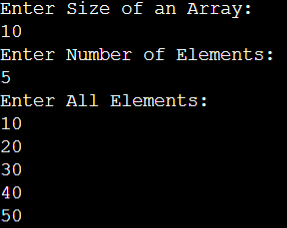Note: In upcoming articles, we will see how we can perform various operations on an array.

In the next article, I am going to discuss How to Insert an Element in an Array with Examples. Here, in this article, I try to explain Array as ADT (Abstract Data Type) in C and C++ Language with Examples and I hope you enjoy this Array as Abstract Data Type article.